## The Ratio Test

Recall that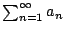is a geometric series if and only if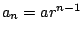for some fixed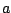and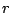. Here we callthe common ratio. Notice that the ratio of any two successive terms is: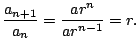Moreover, we have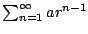converges (to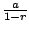) if and only if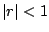(and, of course it diverges if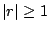).

Example 6.4.5   For example,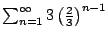converges to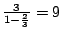. However,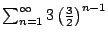diverges.

Theorem 6.4.6 (Ratio Test)   Consider a sum. Then
1. If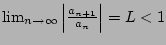thenis absolutely convergent.
2. If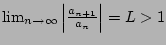thendiverges.
3. If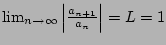then we may conclude nothing from this!

Proof. We will only prove 1. Assume that we have. Let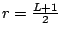, and notice that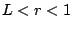(since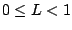, so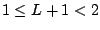, so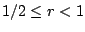, and also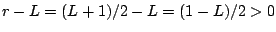).

Since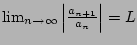, there is an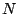such that for all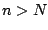we have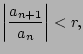so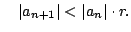Then we have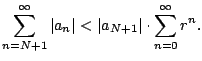Here the common ratio for the second one is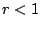, hence thus the right-hand series converges, so the left-hand series converges.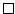Example 6.4.7   Consider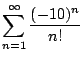. The ratio of successive terms is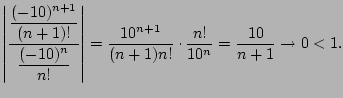Thus this series converges absolutely. Note, the minus sign is missing above since in the ratio test we take the limit of the absolute values.

Example 6.4.8   Consider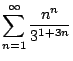. We have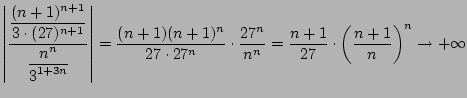Thus our series diverges. (Note here that we use that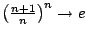.)

Example 6.4.9   Let's apply the ratio test to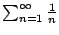. We have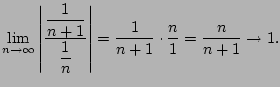This tells us nothing. If this happens... do something else! E.g., in this case, use the integral test.

William Stein 2006-03-15